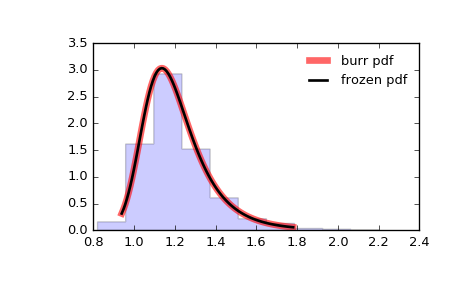# scipy.stats.burr¶

scipy.stats.burr = <scipy.stats._continuous_distns.burr_gen object>[source]

A Burr (Type III) continuous random variable.

As an instance of the rv_continuous class, burr object inherits from it a collection of generic methods (see below for the full list), and completes them with details specific for this particular distribution.

fisk
a special case of either burr or burr12 with d = 1
burr12
Burr Type XII distribution

Notes

The probability density function for burr is:

burr.pdf(x, c, d) = c * d * x**(-c-1) * (1+x**(-c))**(-d-1)


for x > 0.

burr takes c and d as shape parameters.

This is the PDF corresponding to the third CDF given in Burr’s list; specifically, it is equation (11) in Burr’s paper [R561].

The probability density above is defined in the “standardized” form. To shift and/or scale the distribution use the loc and scale parameters. Specifically, burr.pdf(x, c, d, loc, scale) is identically equivalent to burr.pdf(y, c, d) / scale with y = (x - loc) / scale.

References

 [R561] (1, 2) Burr, I. W. “Cumulative frequency functions”, Annals of Mathematical Statistics, 13(2), pp 215-232 (1942).

Examples

>>> from scipy.stats import burr
>>> import matplotlib.pyplot as plt
>>> fig, ax = plt.subplots(1, 1)


Calculate a few first moments:

>>> c, d = 10.5, 4.3
>>> mean, var, skew, kurt = burr.stats(c, d, moments='mvsk')


Display the probability density function (pdf):

>>> x = np.linspace(burr.ppf(0.01, c, d),
...                 burr.ppf(0.99, c, d), 100)
>>> ax.plot(x, burr.pdf(x, c, d),
...        'r-', lw=5, alpha=0.6, label='burr pdf')


Alternatively, the distribution object can be called (as a function) to fix the shape, location and scale parameters. This returns a “frozen” RV object holding the given parameters fixed.

Freeze the distribution and display the frozen pdf:

>>> rv = burr(c, d)
>>> ax.plot(x, rv.pdf(x), 'k-', lw=2, label='frozen pdf')


Check accuracy of cdf and ppf:

>>> vals = burr.ppf([0.001, 0.5, 0.999], c, d)
>>> np.allclose([0.001, 0.5, 0.999], burr.cdf(vals, c, d))
True


Generate random numbers:

>>> r = burr.rvs(c, d, size=1000)


And compare the histogram:

>>> ax.hist(r, normed=True, histtype='stepfilled', alpha=0.2)
>>> ax.legend(loc='best', frameon=False)
>>> plt.show()Methods

 rvs(c, d, loc=0, scale=1, size=1, random_state=None) Random variates. pdf(x, c, d, loc=0, scale=1) Probability density function. logpdf(x, c, d, loc=0, scale=1) Log of the probability density function. cdf(x, c, d, loc=0, scale=1) Cumulative distribution function. logcdf(x, c, d, loc=0, scale=1) Log of the cumulative distribution function. sf(x, c, d, loc=0, scale=1) Survival function (also defined as 1 - cdf, but sf is sometimes more accurate). logsf(x, c, d, loc=0, scale=1) Log of the survival function. ppf(q, c, d, loc=0, scale=1) Percent point function (inverse of cdf — percentiles). isf(q, c, d, loc=0, scale=1) Inverse survival function (inverse of sf). moment(n, c, d, loc=0, scale=1) Non-central moment of order n stats(c, d, loc=0, scale=1, moments='mv') Mean(‘m’), variance(‘v’), skew(‘s’), and/or kurtosis(‘k’). entropy(c, d, loc=0, scale=1) (Differential) entropy of the RV. fit(data, c, d, loc=0, scale=1) Parameter estimates for generic data. expect(func, args=(c, d), loc=0, scale=1, lb=None, ub=None, conditional=False, **kwds) Expected value of a function (of one argument) with respect to the distribution. median(c, d, loc=0, scale=1) Median of the distribution. mean(c, d, loc=0, scale=1) Mean of the distribution. var(c, d, loc=0, scale=1) Variance of the distribution. std(c, d, loc=0, scale=1) Standard deviation of the distribution. interval(alpha, c, d, loc=0, scale=1) Endpoints of the range that contains alpha percent of the distribution